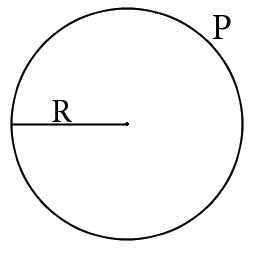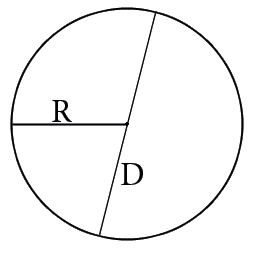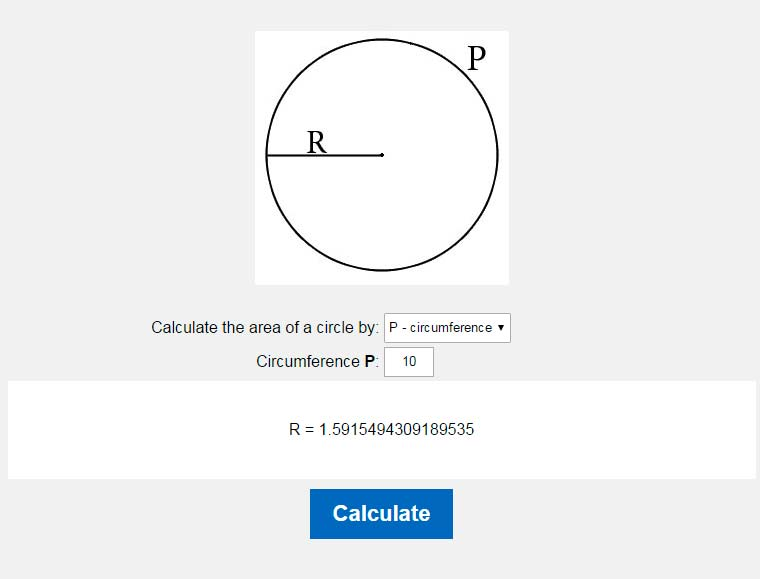# Radius of a circle calculator. Calculate the radius of a circle.

You could easy calculate circle's radius by our online calculator.Calculate the area of a circle by: P - circumferenceS - areaD - diameter Circumference P:

In order to calculate the radius of the circle must know the length or area. If we know one of the ukazannh values will not be difficult to calculate the radius of the circle for us.
The circle radius is calculated as follows:

1. If we know the length:
The formula for calculating the radius of the circle through its length:
R=P/(2π)2. If we know the area:
The formula for calculating the radius of the circle through the area:
R=S/π3. If we know the diameter:
The formula for calculating the radius of the circle through diameter:
R=D/2Where R - radius of the circle, S - area of a circle, P - the circle length, D - diameter, π - Pi is always approximately equal to 3.14.

### The radius of the circle through its length### The radius of the circle through the area### The radius of the circle through diameterHOME
RESOURCES